###### python操作Excel神器openpyxl的各种操作！
2020-09-29openpyxl是一个非标准库，因此需要自行安装，安装过程并不困难，Windows/Mac用户均可以在命令行(CMD)/终端(Terminal)中使用pip安装

`pip install openpyxl`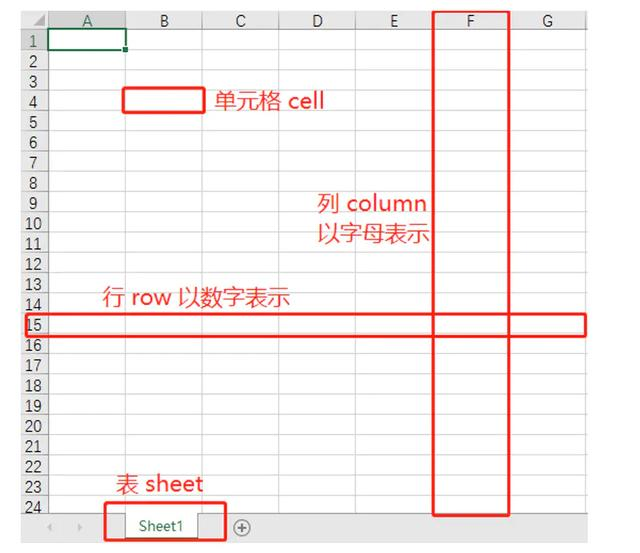1.载入Excel

```from openpyxl import load_workbook
print(workbook.sheetnames)```

2.根据名称获取工作表

```from openpyxl import load_workbook
print(workbook.sheetnames)
sheet = workbook['工作业务']```

`sheet = workbook.active`

3.获取表格内容所在的范围

`print(sheet.dimensions)`

4.获取某个单元格的具体内容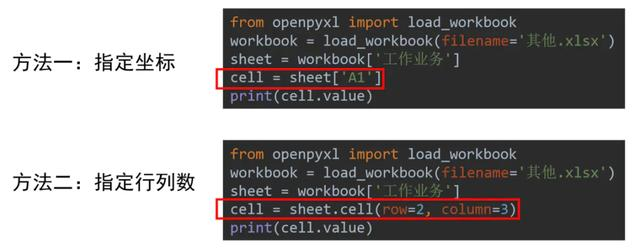5.获取某个单元格的行、列、坐标

`print(cell.row, cell.column, cell.coordinate)`

6.获取多个格子的值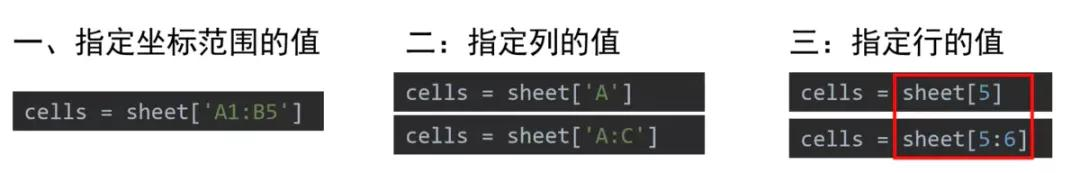```for cell in cells:
print(cell.value)```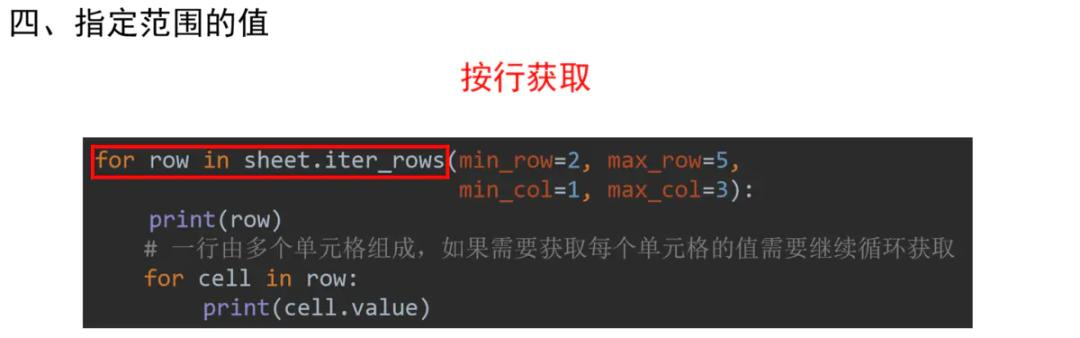7.读取所有的行

```for row in sheet.rows:
print(row)```

Excel写入

1. 保存Excel

`workbook.save(filename='Excel工作表1.xlsx')`
• 如果读取和写入Excel的路径相同则为对原文件进行修改
• 如果读取和写入Excel的路径不同则为保存成新的文件

2.写入单元格

```cell = sheet['A1']
cell.value = '业务需求```

3.写入一行或多行数据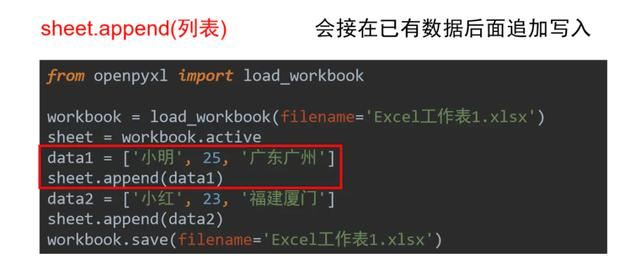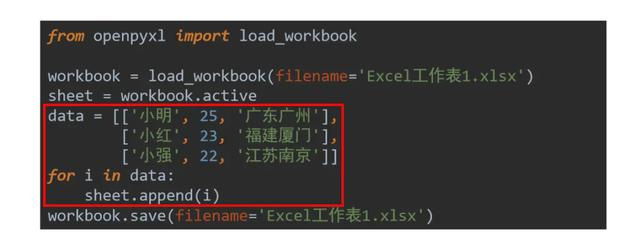4.将公式写入单元格并保存

`sheet['K11'] = '=AVERAGE(K1:K10)' `

5.插入一行或多行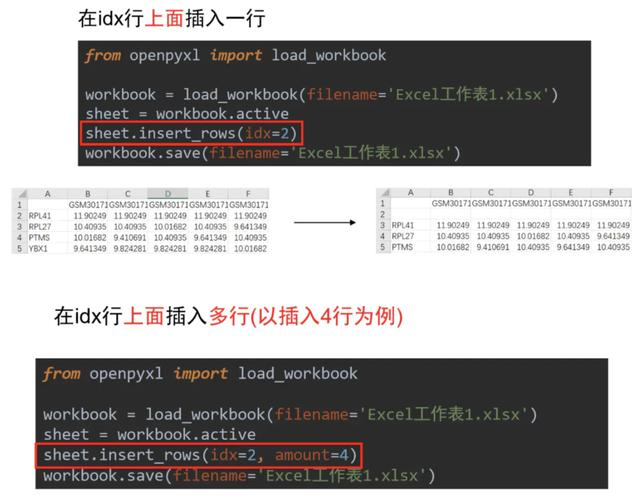6. 插入一列或多列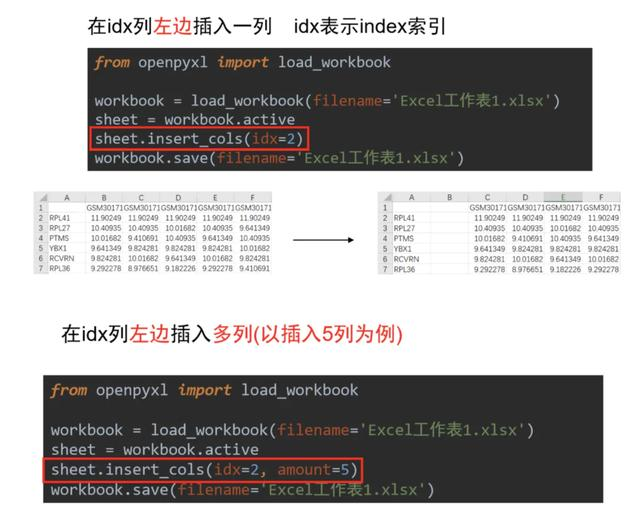7.删除多行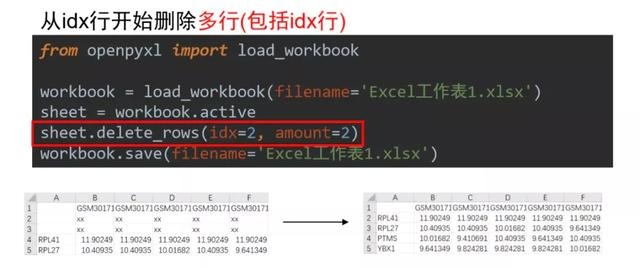8.删除多列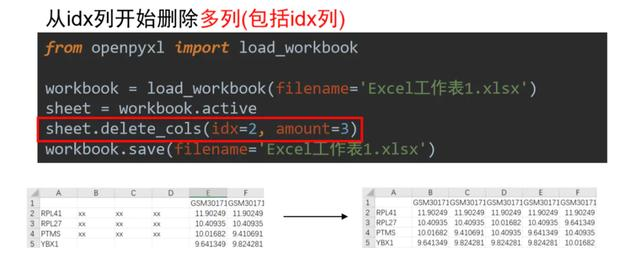9. 移动范围数据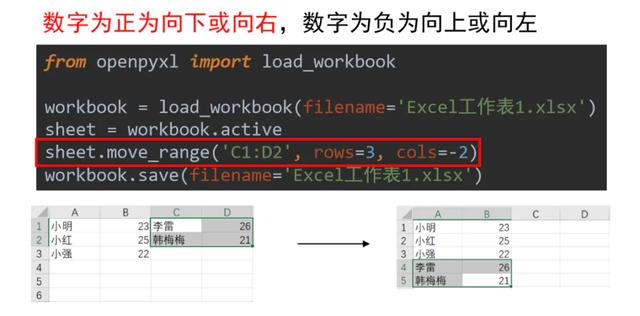10. 创建新的Excel表格

```from openpyxl import Workbook
workbook = Workbook()```

Excel样式调整

1. 设置字体样式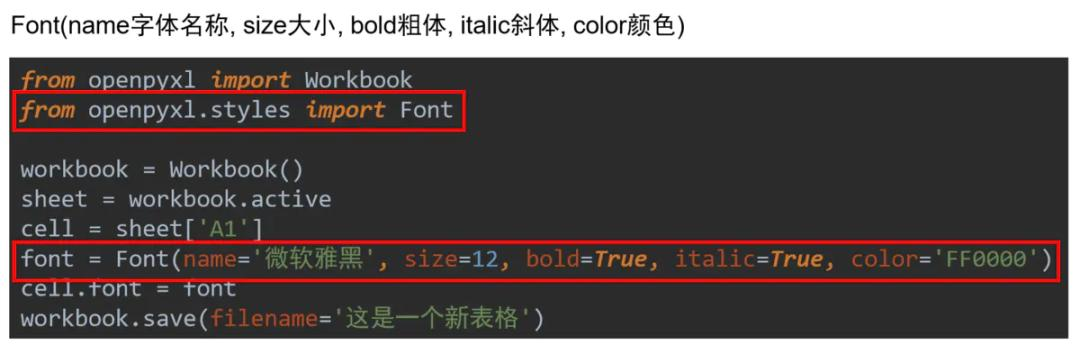2. 设置对齐样式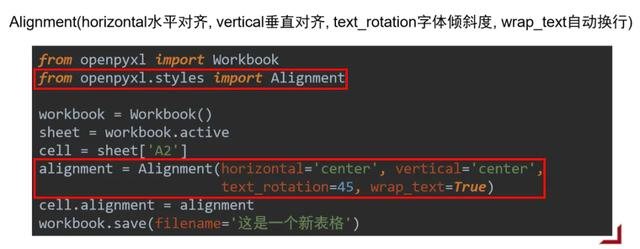• 水平对齐:distributed, justify, center, left, fill, centerContinuous, right, general
• 垂直对齐:bottom, distributed, justify, center, top

3. 设置边框样式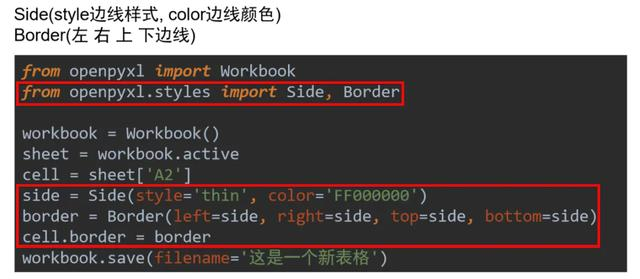• 边线样式:double, mediumDashDotDot, slantDashDot, dashDotDot, dotted, hair, mediumDashed, dashed, dashDot, thin, mediumDashDot, medium, thick

4. 设置单元格填充样式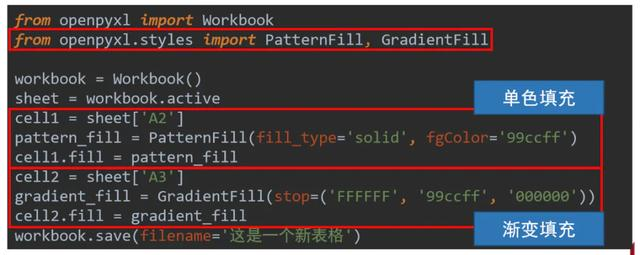5. 设置行高和列宽

```sheet.row_dimensions.height = 50
sheet.column_dimensions['C'].width = 20 ```

6. 单元格合并与取消

```# 合并
sheet.merge_cells('A1:B2')
sheet.merge_cells(start_row=1, start_column=3,
end_row=2, end_column=4)

# 取消合并
sheet.unmerge_cells('A1:B2')
sheet.unmerge_cells(start_row=1, start_column=3,
end_row=2, end_column=4)```

```
想从事业务型数据分析师，您可以点击>>>“数据分析师”了解课程详情；

```